## Introduction

My 5-qubit Quantum Computing Simulator,QCSimulator, is finally ready, and here it is! I have been able to successfully complete this simulator by working through a fair amount of material. To a large extent, the simulator is easy, if one understands how to solve the quantum circuit. However the theory behind computing itself, is quite formidable and I hope to scale this mountain over a period of time.

As of now the R package can be installed from Github. I have to complete some documentation and tie a couple of loose ends before it gets onto CRAN.

The code for the QCSimulator package is available at Github QCSimulator. This post has also been published at Rpubs as QCSimulator and can be downloaded as a PDF document at QCSimulator.pdf

Disclaimer: This article represents the author’s viewpoint only and doesn’t necessarily represent IBM’s positions, strategies or opinions

library(QCSimulator)
library(ggplot2)

### 1. Initialize the environment and set global variables

Here I initialize the environment with global variables and then display a few of them.

rm(list=ls())
#Call the init function to initialize the environment and create global variables
init()

# Display some of global variables in environment
ls()
##   "I16"     "I2"      "I4"      "I8"      "q0_"     "q00_"    "q000_"
##   "q0000_"  "q00000_" "q00001_" "q0001_"  "q00010_" "q00011_" "q001_"
##  "q0010_"  "q00100_" "q00101_" "q0011_"  "q00110_" "q00111_" "q01_"
##  "q010_"   "q0100_"  "q01000_" "q01001_" "q0101_"  "q01010_" "q01011_"
##  "q011_"   "q0110_"  "q01100_" "q01101_" "q0111_"  "q01111_" "q1_"
##  "q10_"    "q100_"   "q1000_"  "q10000_" "q10001_" "q1001_"  "q10010_"
##  "q10011_" "q101_"   "q1010_"  "q10100_" "q10101_" "q1011_"  "q10110_"
##  "q10111_" "q11_"    "q110_"   "q1100_"  "q11000_" "q11001_" "q1101_"
##  "q11010_" "q11011_" "q111_"   "q1110_"  "q11100_" "q11101_" "q1111_"
##  "q11110_" "q11111_"
#1. 2 x 2 Identity matrix
I2
##      [,1] [,2]
## [1,]    1    0
## [2,]    0    1
#2. 8 x 8 Identity matrix
I8
##      [,1] [,2] [,3] [,4] [,5] [,6] [,7] [,8]
## [1,]    1    0    0    0    0    0    0    0
## [2,]    0    1    0    0    0    0    0    0
## [3,]    0    0    1    0    0    0    0    0
## [4,]    0    0    0    1    0    0    0    0
## [5,]    0    0    0    0    1    0    0    0
## [6,]    0    0    0    0    0    1    0    0
## [7,]    0    0    0    0    0    0    1    0
## [8,]    0    0    0    0    0    0    0    1
#3. Qubit |00>
q00_
##      [,1]
## [1,]    1
## [2,]    0
## [3,]    0
## [4,]    0
#4. Qubit |010>
q010_
##      [,1]
## [1,]    0
## [2,]    0
## [3,]    1
## [4,]    0
## [5,]    0
## [6,]    0
## [7,]    0
## [8,]    0
#5. Qubit |0100>
q0100_
##       [,1]
##  [1,]    0
##  [2,]    0
##  [3,]    0
##  [4,]    0
##  [5,]    1
##  [6,]    0
##  [7,]    0
##  [8,]    0
##  [9,]    0
## [10,]    0
## [11,]    0
## [12,]    0
## [13,]    0
## [14,]    0
## [15,]    0
## [16,]    0
#6. Qubit 10010
q10010_
##       [,1]
##  [1,]    0
##  [2,]    0
##  [3,]    0
##  [4,]    0
##  [5,]    0
##  [6,]    0
##  [7,]    0
##  [8,]    0
##  [9,]    0
## [10,]    0
## [11,]    0
## [12,]    0
## [13,]    0
## [14,]    0
## [15,]    0
## [16,]    0
## [17,]    0
## [18,]    0
## [19,]    1
## [20,]    0
## [21,]    0
## [22,]    0
## [23,]    0
## [24,]    0
## [25,]    0
## [26,]    0
## [27,]    0
## [28,]    0
## [29,]    0
## [30,]    0
## [31,]    0
## [32,]    0

The QCSimulator implements the following gates

1. Pauli X,Y,Z, S,S’, T, T’ gates
3. 2,3,4,5 qubit CNOT gates e.g CNOT2_01,CNOT3_20,CNOT4_13 etc
4. Toffoli State,SWAPQ0Q1

### 2. To display the unitary matrix of gates

To check the unitary matrix of gates, we need to pass the appropriate identity matrix as an argument. Hence below the qubit gates require a 2 x 2 unitary matrix and the 2 & 3 qubit CNOT gates require a 4 x 4 and 8 x 8 identity matrix respectively

PauliX(I2)
##      [,1] [,2]
## [1,]    0    1
## [2,]    1    0
Hadamard(I2)
##           [,1]       [,2]
## [1,] 0.7071068  0.7071068
## [2,] 0.7071068 -0.7071068
S1Gate(I2)
##      [,1] [,2]
## [1,] 1+0i 0+0i
## [2,] 0+0i 0-1i
CNOT2_10(I4)
##      [,1] [,2] [,3] [,4]
## [1,]    1    0    0    0
## [2,]    0    0    0    1
## [3,]    0    0    1    0
## [4,]    0    1    0    0
CNOT3_20(I8)
##      [,1] [,2] [,3] [,4] [,5] [,6] [,7] [,8]
## [1,]    1    0    0    0    0    0    0    0
## [2,]    0    0    0    0    0    1    0    0
## [3,]    0    0    1    0    0    0    0    0
## [4,]    0    0    0    0    0    0    0    1
## [5,]    0    0    0    0    1    0    0    0
## [6,]    0    1    0    0    0    0    0    0
## [7,]    0    0    0    0    0    0    1    0
## [8,]    0    0    0    1    0    0    0    0

### 3. Compute the inner product of vectors

For example of phi = 1/2|0> + sqrt(3)/2|1> and si= 1/sqrt(2)(10> + |1>) then the inner product is the dot product of the vectors

phi = matrix(c(1/2,sqrt(3)/2),nrow=2,ncol=1)
si = matrix(c(1/sqrt(2),1/sqrt(2)),nrow=2,ncol=1)
angle= innerProduct(phi,si)
cat("Angle between vectors is:",angle)
## Angle between vectors is: 15

### 4. Compute the dagger function for a gate

The gate dagger computes and displays the transpose of the complex conjugate of the matrix

TGate(I2)
##      [,1]                 [,2]
## [1,] 1+0i 0.0000000+0.0000000i
## [2,] 0+0i 0.7071068+0.7071068i
GateDagger(TGate(I2))
##      [,1]                 [,2]
## [1,] 1+0i 0.0000000+0.0000000i
## [2,] 0+0i 0.7071068-0.7071068i

### 5. To display the unitary matrix of gates

To check the unitary matrix of gates we need to pass the appropriate identity matrix. Hence below the qubit gates require a 2 x 2 unitary matrix and the 2 & 3 qubit CNOT gates require a 4 x 4 and 8 x 8 identity matrix respectively.

PauliX(I2)
##      [,1] [,2]
## [1,]    0    1
## [2,]    1    0
Hadamard(I2)
##           [,1]       [,2]
## [1,] 0.7071068  0.7071068
## [2,] 0.7071068 -0.7071068
S1Gate(I2)
##      [,1] [,2]
## [1,] 1+0i 0+0i
## [2,] 0+0i 0-1i
CNOT2_10(I4)
##      [,1] [,2] [,3] [,4]
## [1,]    1    0    0    0
## [2,]    0    0    0    1
## [3,]    0    0    1    0
## [4,]    0    1    0    0
CNOT3_20(I8)
##      [,1] [,2] [,3] [,4] [,5] [,6] [,7] [,8]
## [1,]    1    0    0    0    0    0    0    0
## [2,]    0    0    0    0    0    1    0    0
## [3,]    0    0    1    0    0    0    0    0
## [4,]    0    0    0    0    0    0    0    1
## [5,]    0    0    0    0    1    0    0    0
## [6,]    0    1    0    0    0    0    0    0
## [7,]    0    0    0    0    0    0    1    0
## [8,]    0    0    0    1    0    0    0    0

### 6. Invoking gates in series

The Quantum gates can be chained by passing each preceding Quantum gate as the argument. The final gate in the chain will have the qubit or the identity matrix passed to it.

# Call in reverse order
# Superposition states
# |+> state
Hadamard(q0_)
##           [,1]
## [1,] 0.7071068
## [2,] 0.7071068
# |-> ==> H x Z
PauliZ(Hadamard(q0_))
##            [,1]
## [1,]  0.7071068
## [2,] -0.7071068
# (+i) Y ==> H x  S
SGate(Hadamard(q0_))
##                      [,1]
## [1,] 0.7071068+0.0000000i
## [2,] 0.0000000+0.7071068i
# (-i)Y ==> H x S1
S1Gate(Hadamard(q0_))
##                      [,1]
## [1,] 0.7071068+0.0000000i
## [2,] 0.0000000-0.7071068i
# Q1 -- TGate- Hadamard
Q1 = Hadamard(TGate(I2))

### 7. More gates in series

#### TGate of depth 2

The Quantum circuit for a TGate of Depth 2 is

Q0 — Hadamard-TGate-Hadamard-TGate-SGate-Measurement Implementing the above quantum circuit in QCSimulator we get

# Invoking this in reverse order we get
result=measurement(a)

plotMeasurement(result)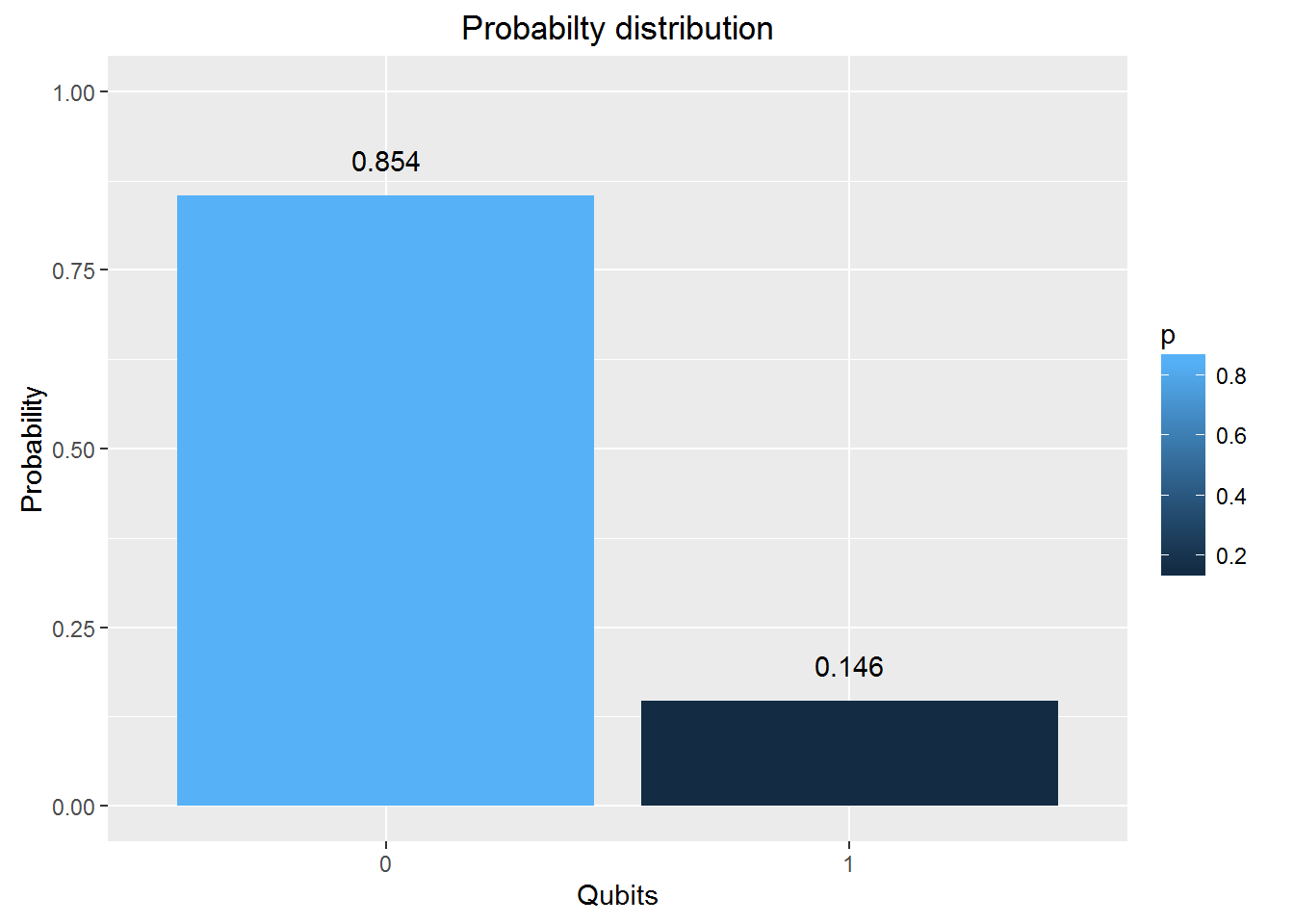### 8. Invoking gates in parallel

To obtain the results of gates in parallel we have to take the Tensor Product Note:In the TensorProduct invocation the Identity matrix is passed as an argument to get the unitary matrix of the gate. Q0 – Hadamard-Measurement Q1 – Identity- Measurement

#
b = DotProduct(a,q00_)
plotMeasurement(measurement(b))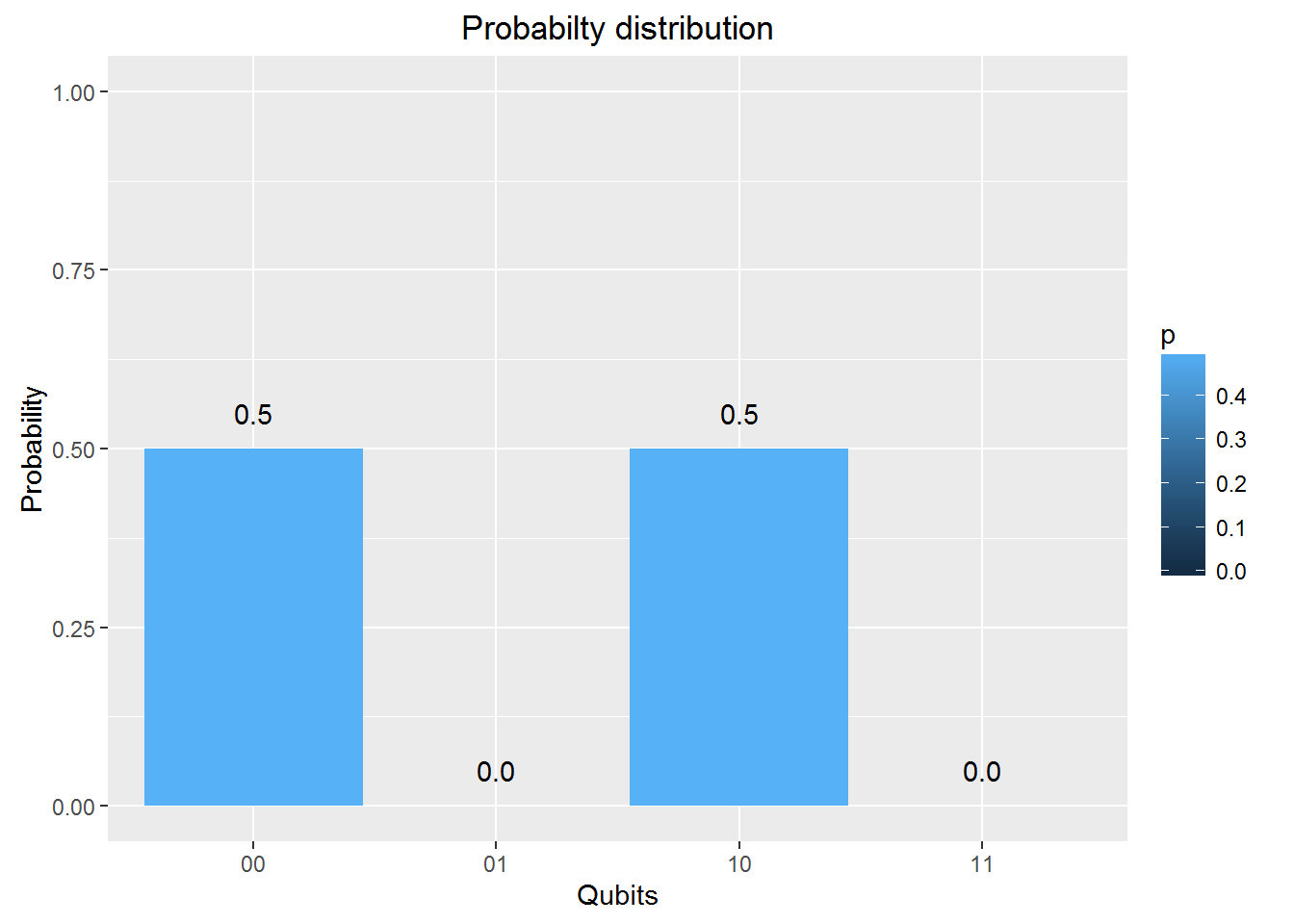a = TensorProd(PauliZ(I2),Hadamard(I2))
b = DotProduct(a,q00_)
plotMeasurement(measurement(b))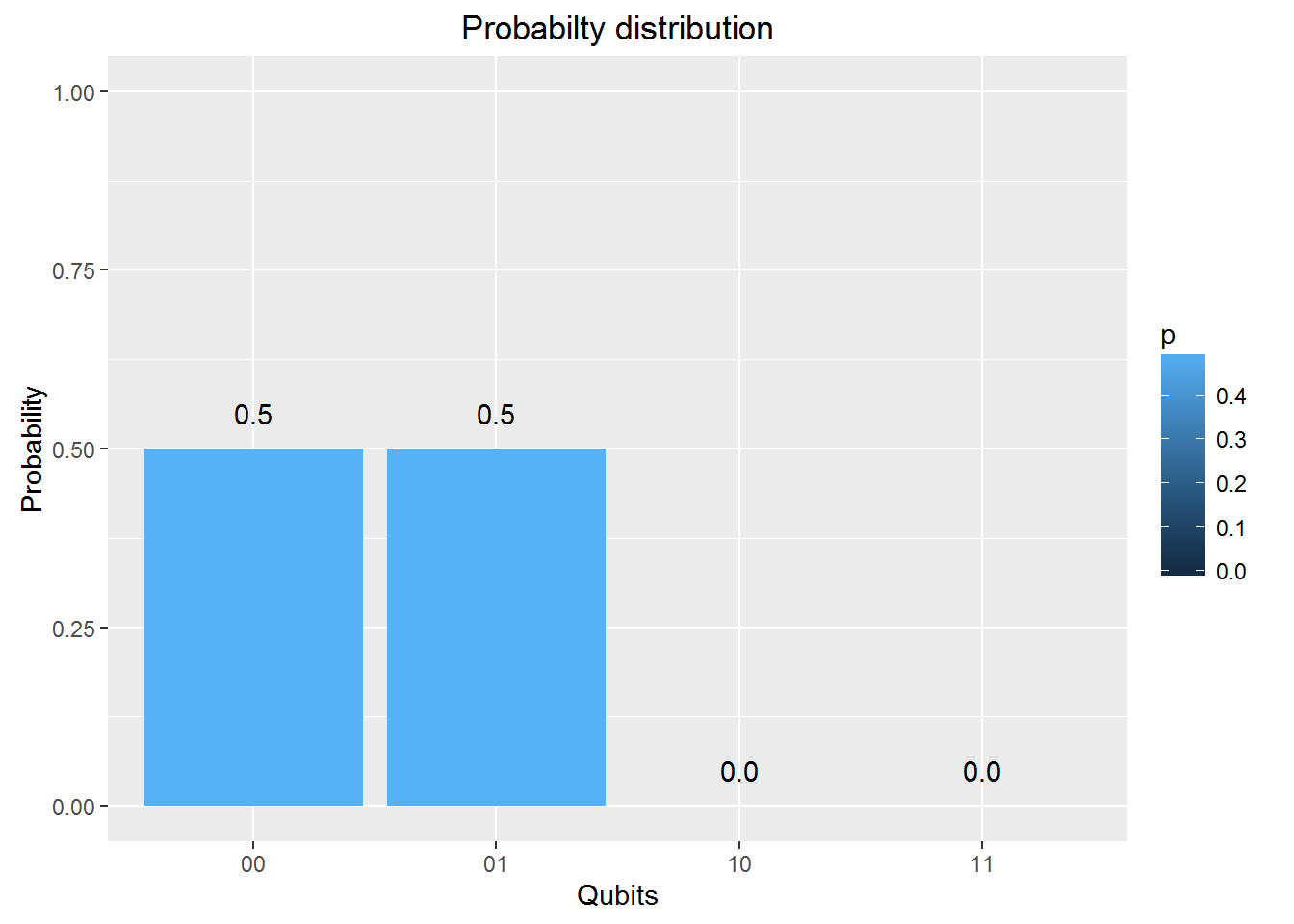### 9. Measurement

The measurement of a Quantum circuit can be obtained using the measurement function. Consider the following Quantum circuit

Q0 - H-T-H-T-S-H-T-H-T-H-T-H-S-Measurement where H - Hadamard gate, T - T Gate and S- S Gate

a = SGate(Hadamard(TGate(Hadamard(TGate(Hadamard(TGate(Hadamard(SGate(TGate(Hadamard(TGate(Hadamard(I2)))))))))))))
measurement(a)
##          0        1
## v 0.890165 0.109835

### 10. Plot measurement

Using the same example as above Q0 - H-T-H-T-S-H-T-H-T-H-T-H-S-Measurement where H - Hadamard gate, T - T Gate and S- S Gate we can plot the measurement

a = SGate(Hadamard(TGate(Hadamard(TGate(Hadamard(TGate(Hadamard(SGate(TGate(Hadamard(TGate(Hadamard(I2)))))))))))))
result = measurement(a)
plotMeasurement(result)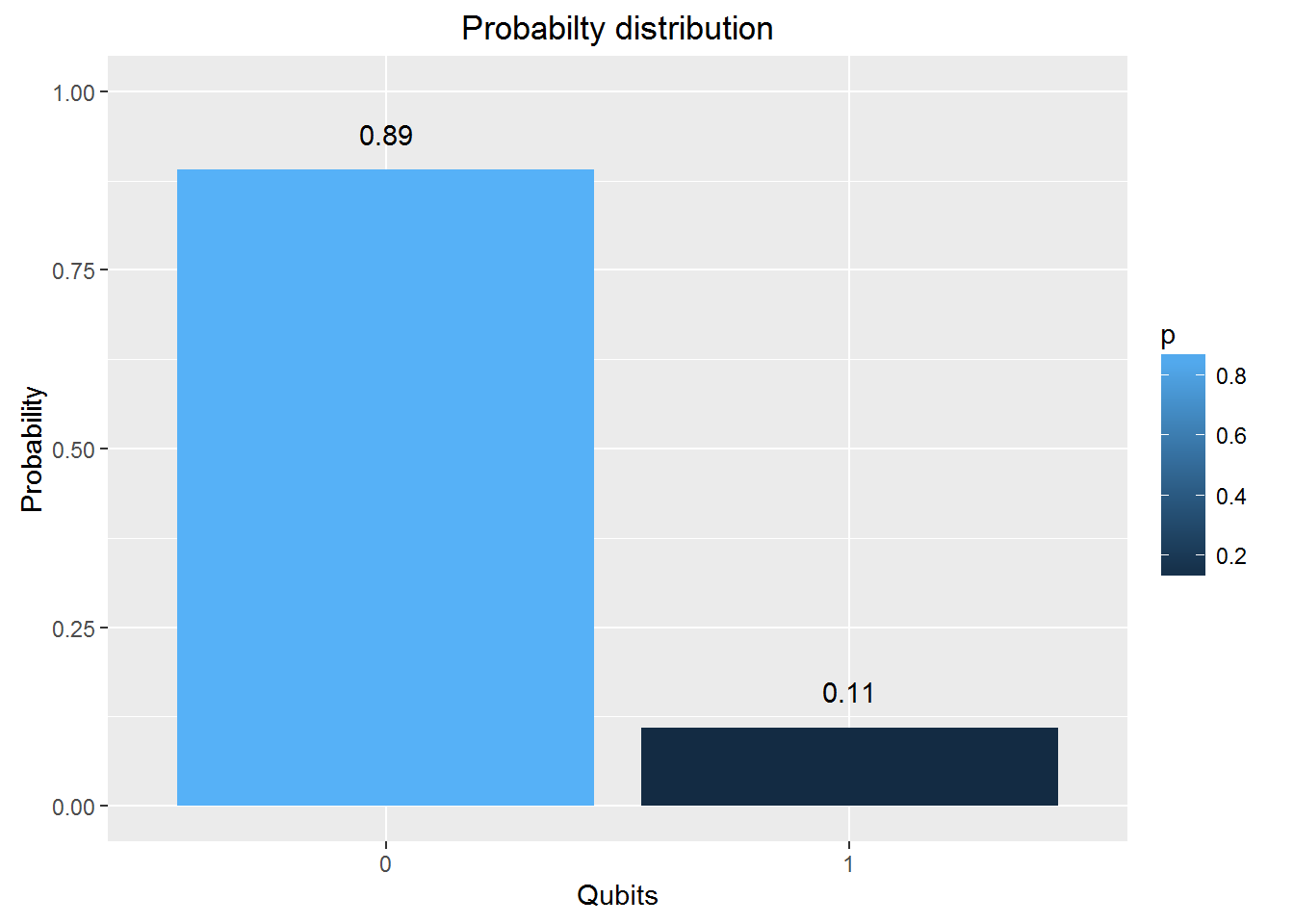### 11. Evaluating a Quantum Circuit

The above procedures for evaluating a quantum gates in series and parallel can be used to evalute more complex quantum circuits where the quantum gates are in series and in parallel.

Here is an evaluation of one such circuit, the Bell ZQ state using the QCSimulator

# 1st composite
# Output of CNOT
b = CNOT2_01(a)
# 2nd series
#3rd composite
d= TensorProd(I2,c)
# Output of 2nd composite
e = DotProduct(b,d)
#Action of quantum circuit on |00>
f = DotProduct(e,q00_)
result= measurement(f)
plotMeasurement(result)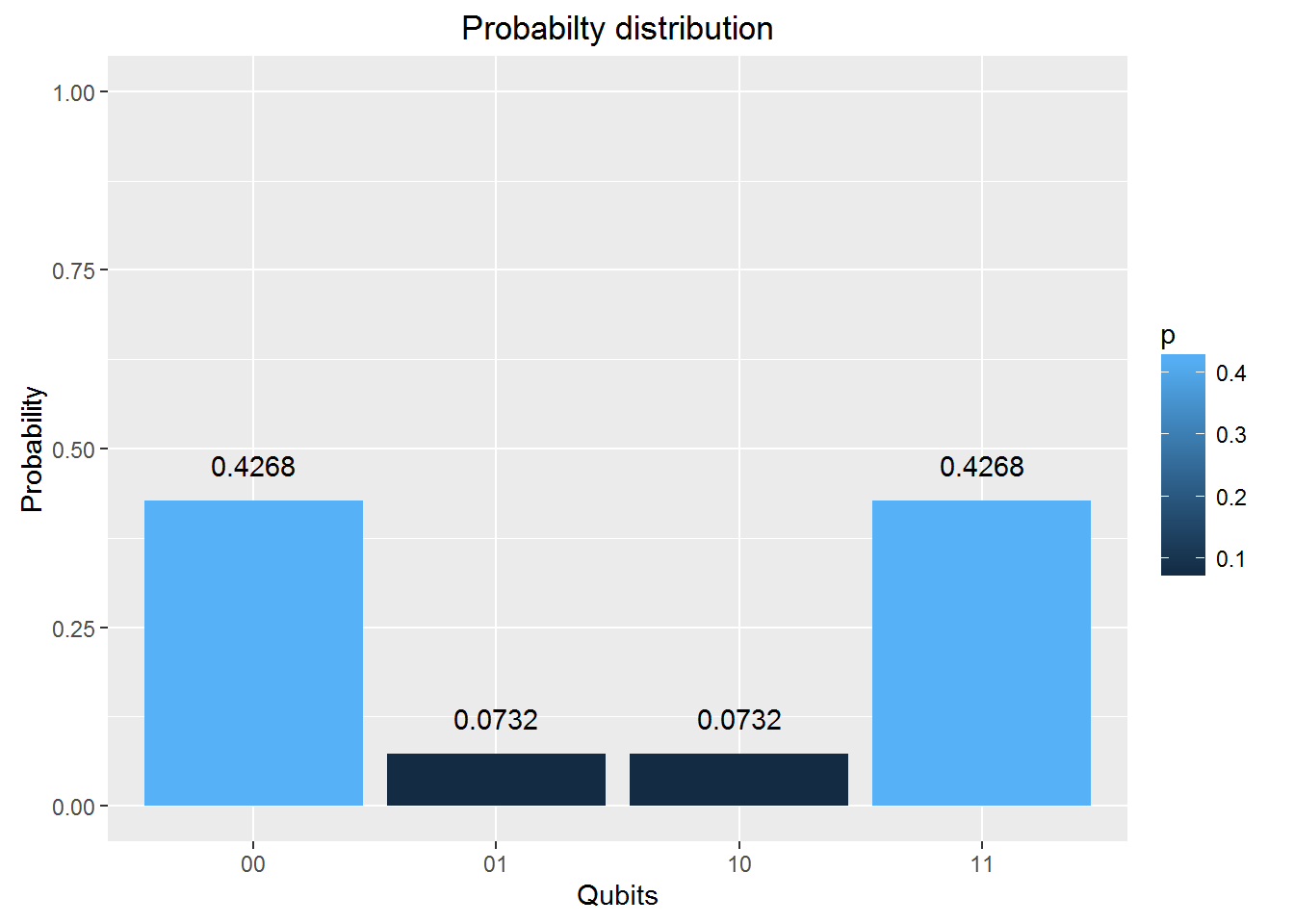### 12. Toffoli State

This circuit for this comes from IBM’s Quantum Experience. This circuit is available in the package. This is how the state was constructed

  # Computation of the Toffoli State
H=1/sqrt(2) * matrix(c(1,1,1,-1),nrow=2,ncol=2)
I=matrix(c(1,0,0,1),nrow=2,ncol=2)

# 1st composite
# H x H x H
a = TensorProd(TensorProd(H,H),H)
# 1st CNOT
a1= CNOT3_12(a)

# 2nd composite
# I x I x T1Gate
b = TensorProd(TensorProd(I,I),T1Gate(I))
b1 = DotProduct(b,a1)
c = CNOT3_02(b1)

# 3rd composite
# I x I x TGate
d = TensorProd(TensorProd(I,I),TGate(I))
d1 = DotProduct(d,c)
e = CNOT3_12(d1)

# 4th composite
# I x I x T1Gate
f = TensorProd(TensorProd(I,I),T1Gate(I))
f1 = DotProduct(f,e)
g = CNOT3_02(f1)

#5th composite
# I x T x T
h = TensorProd(TensorProd(I,TGate(I)),TGate(I))
h1 = DotProduct(h,g)
i = CNOT3_12(h1)

#6th composite
# I x H x H
j1 = DotProduct(j,i)
k = CNOT3_12(j1)

# 7th composite
# I x H x H
l1 = DotProduct(l,k)
m = CNOT3_12(l1)
n = CNOT3_02(m)

#8th composite
# T x H x T1
o1 = DotProduct(o,n)
p = CNOT3_02(o1)
result = measurement(p)
plotMeasurement(result)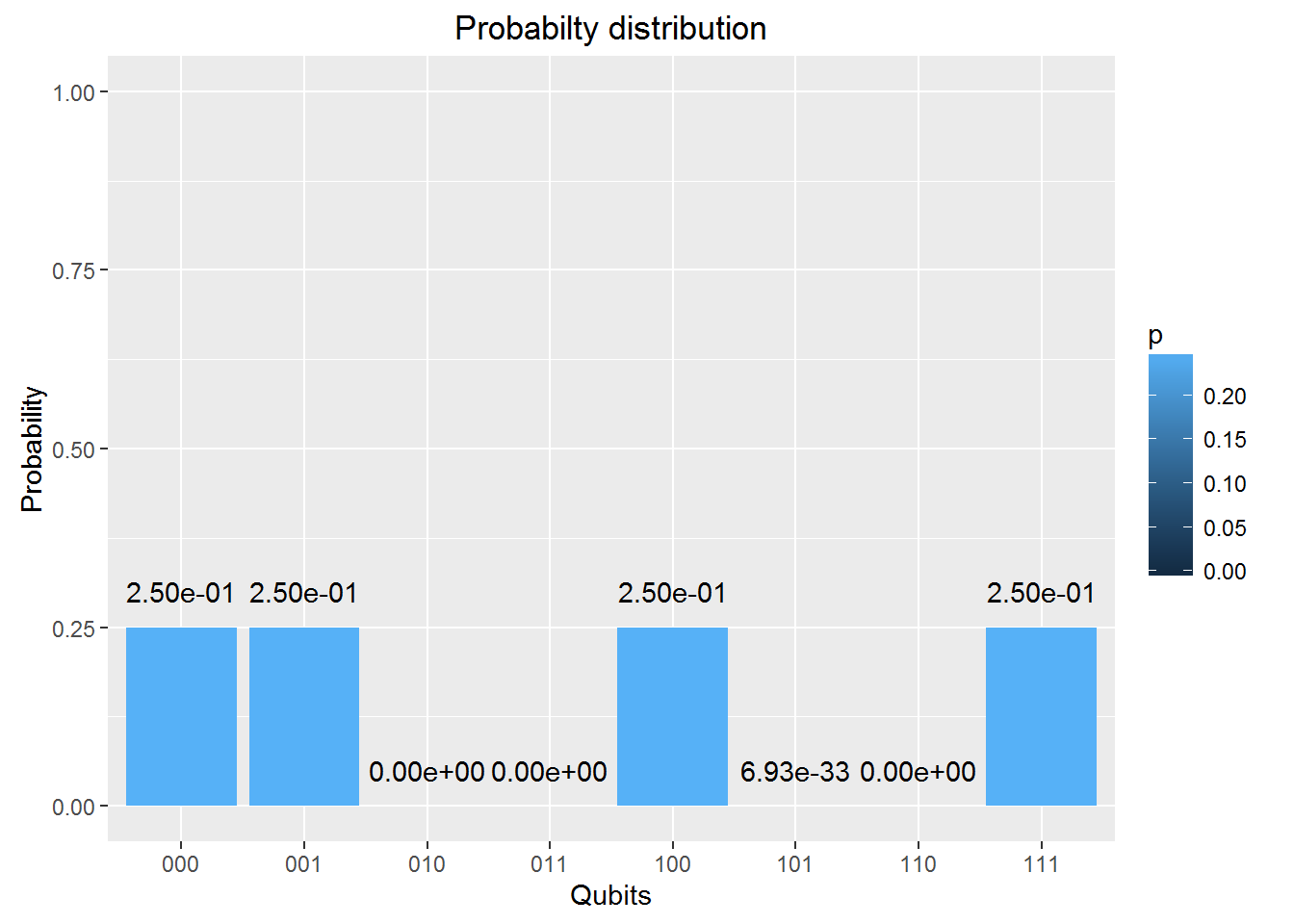### 13. GHZ YYX measurement

Here is another Quantum circuit, namely the entangled GHZ YYX state

# Composite 1
b= CNOT3_12(a)
c= CNOT3_02(b)
# Composite 2
e= DotProduct(d,c)
#Composite 3
g= DotProduct(f,e)
#Composite 4
j = DotProduct(i,g)
result=measurement(j)
plotMeasurement(result)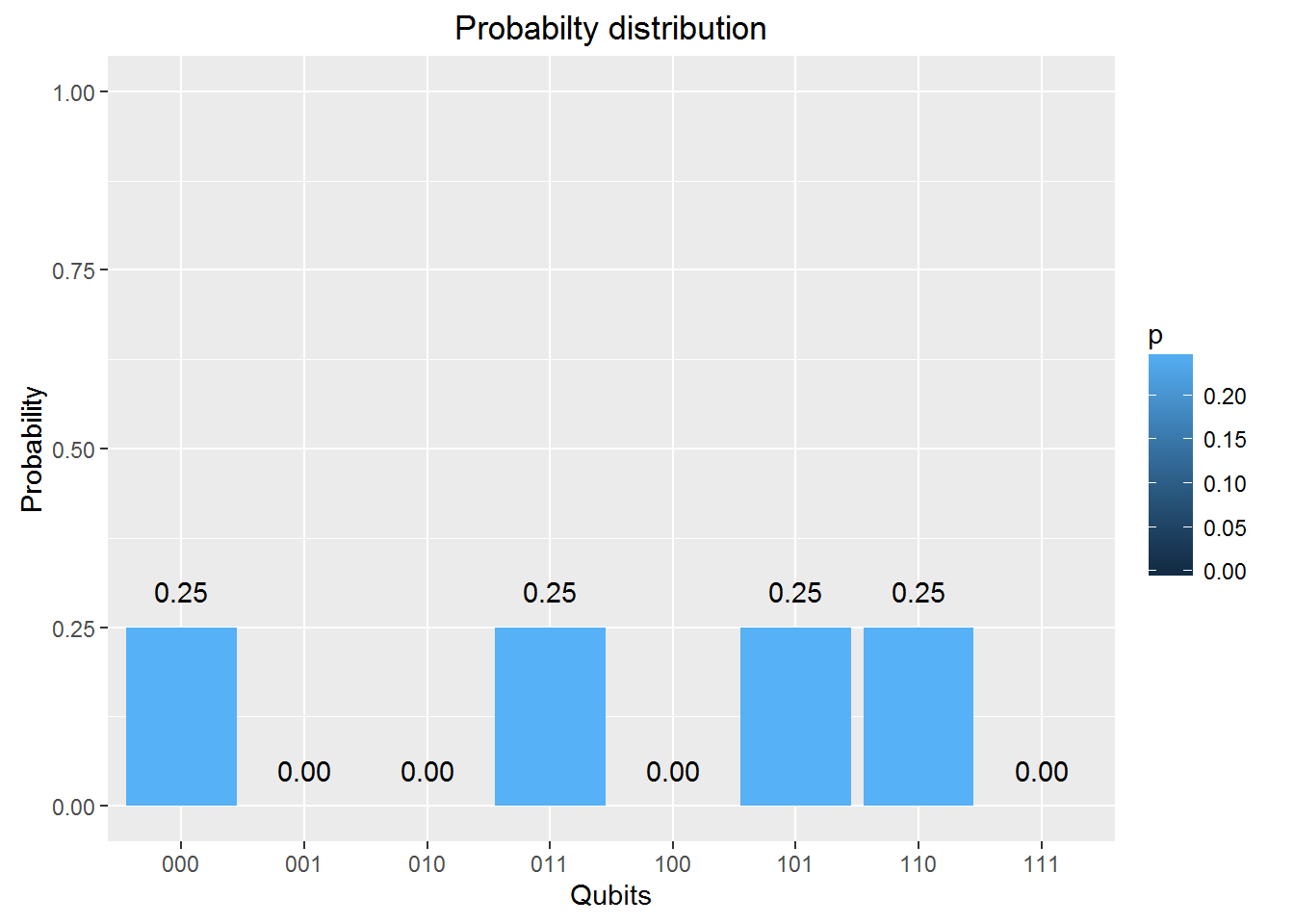## Conclusion

The 5 qubit Quantum Computing Simulator is now fully functional. I hope to add more gates and functionality in the months to come.

Feel free to install the package from Github and give it a try.

Disclaimer: This article represents the author’s viewpoint only and doesn’t necessarily represent IBM’s positions, strategies or opinions

References

Also see

For more posts on other topics like Cloud Computing, IBM Bluemix, Distributed Computing, OpenCV, R, cricket please check my Index of posts